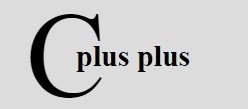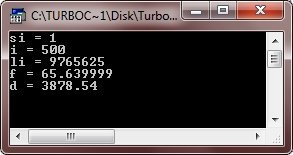# C++ Numbers

Numbers play an important role in almost every program in C++. In general, we uses numbers to perform calculations. Let's see how to define, assign, and then prints numbers in C++

## C++ Number Definition

Here is an example, defining some numbers in C++

```short int si;
int i;
long int li;
float f;
double d;```

## C++ Number Assignments

Here is an example, assigning some values (numbers) to the above defined variables

```si = 1;
i = 500;
li = 9765625;
f = 65.64;
d = 3878.54;```

## C++ Number Printing

Here is an example, printing all the numbers, defined and assigned above:

```cout<<"si = "<<si<<endl;
cout<<"i = "<<i<<endl;
cout<<"li = "<<li<<endl;
cout<<"f = "<<f<<endl;
cout<<"d = "<<d<<endl;```

## C++ Numbers Example

Here is the complete version program of the above provided examples

```/* C++ Numbers */

#include<iostream.h>
#include<conio.h>
void main()
{
clrscr();

short int si;
int i;
long int li;
float f;
double d;

si = 1;
i = 500;
li = 9765625;
f = 65.64;
d = 3878.54;

cout<<"si = "<<si<<endl;
cout<<"i = "<<i<<endl;
cout<<"li = "<<li<<endl;
cout<<"f = "<<f<<endl;
cout<<"d = "<<d<<endl;

getch();
}```

Here is the sample output of the above C++ program:### More Examples

Here are some more C++ programs listed including number conversion and random number printing programs, that you may like:

Tools
Calculator